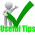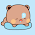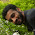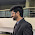Wednesday, January 6, 2010

Minimum Spanning Tree, Kruskal's Algorithm

Kruskal's algorithm is an algorithm in graph theory that finds a minimum spanning tree for a connected weighted undirected graph. This means it finds a subset of the edges that forms a tree that includes every vertex, where the total weight of all the edges in the tree is minimized. If the graph is not connected, then it finds a minimum spanning forest (a minimum spanning tree for each connected component). This algorithm is based on greedy approach.

Performance

This algorithm needs to sort the edges and uses a disjoint set data structure to keep track which vertex is in which component. We know the best comparison sorting is O(e*lg(e)), i.e. the merge sort or quick sort, where e is the number of edges, and the set operations can be implemented such a way that they are almost constant. The algorithm itself is linear to the number of edges e. So the total complexity can be achieved is O(e*lg(e)). Also note that, e can be max v*v (when it is a complete graph).

Algorithm

Do some study and paper-work before you proceed:
1. the algorithm and analysis
2. another good pseudo-code
4. text: Introduction to Algorithms (CLRS, MIT press), Chapter 23.

Implementation

Here is a short and in general implementation in C++ using the STL library. This solves for one graph, if you need it for multiple graphs inputs, don't forget to reset the vectors and arrays appropriately.

Input

N, E // number of nodes and edges.
E edges containing u, v, w; where, the edge is (u, v) and edge weight is w.

C++ code

`#include <cstdio>#include <vector>#include <algorithm>using namespace std;#define edge pair< int, int >#define MAX 1001// ( w (u, v) ) formatvector< pair< int, edge > > GRAPH, MST;int parent[MAX], total, N, E;int findset(int x, int *parent){    if(x != parent[x])        parent[x] = findset(parent[x], parent);    return parent[x];}void kruskal(){    int i, pu, pv;    sort(GRAPH.begin(), GRAPH.end()); // increasing weight    for(i=total=0; i<E; i++)    {        pu = findset(GRAPH[i].second.first, parent);        pv = findset(GRAPH[i].second.second, parent);        if(pu != pv)        {            MST.push_back(GRAPH[i]); // add to tree            total += GRAPH[i].first; // add edge cost            parent[pu] = parent[pv]; // link        }    }}void reset(){    // reset appropriate variables here    // like MST.clear(), GRAPH.clear(); etc etc.    for(int i=1; i<=N; i++) parent[i] = i;}void print(){    int i, sz;    // this is just style...    sz = MST.size();    for(i=0; i<sz; i++)    {        printf("( %d", MST[i].second.first);        printf(", %d )", MST[i].second.second);        printf(": %d\n", MST[i].first);    }    printf("Minimum cost: %d\n", total);}int main(){    int i, u, v, w;    scanf("%d %d", &N, &E);    reset();    for(i=0; i<E; i++)    {        scanf("%d %d %d", &u, &v, &w);        GRAPH.push_back(pair< int, edge >(w, edge(u, v)));    }    kruskal(); // runs kruskal and construct MST vector    print(); // prints MST edges and weights    return 0;}`

Have fun and please notify for any bug...

1.Could u plz explain why setting parent[pu] = parent[pv] successfully detects cycle ?? done a pretty decent paper work...and understands why..but still need a solid theorem.

2.@Munna, here is the theory...

3.Impressive code. This is the cleanest implementation I have seen of Kruskal's to date. It sticks very close to the pseudo-code -- I like it!

4.Yeah, I also don't find any reason why people can't help writing some oop mumbojumbo's whenever they come to write something over the net. The do nothing but make algorithms harder to follow...

5.6.I can't understand what are you trying to indicate by "doing an input", are you asking "how to take input"? Well, it's a language dependent issue and you can always use google!

7.Thanks!, a nice implementation of Kruskal.
I would remove the recursive findset() to get it even better

int findset(int x, int* parent)
{
while(x!= parent[x])
x=parent[x];
return x;
}

8.@Anonymous, are you sure you are doing the right thing? your findset does not do anything the recursive one does. In findset, you are supposed to update parent[] that's why it is called path compression. But clearly, you have increased the complexity to O(n) and your algorithm will not even work properly. Let me know if it does.

9.Removing recursion does not always get things better, at least not the way you have mentioned. Check this, and see if you can get 100:
http://www.spoj.pl/problems/MST/

10.It will work the same. In findset() we are just finding out the disjoint set it belonging to, we are not updating it.Updating the parent is in Kruskal().
Here is result of that problem:
4<----->2:2
1<----->3:5
1<----->2:10
Total distance=17

I think complexity and the number of terms computed in both cases remains the same.

11."we are not updating it." you are wrong, and I think you need to have some study on path compression. the findset definitely updates parent. and recursive one presented here takes constant time max O(4) while yours one is linear O(n). so, before running with kruskal, first finish this http://www.topcoder.com/tc?module=Static&d1=tutorials&d2=disjointDataStructure
Thanks

12.One more thing, I was not aware of the spoj website, thanks for it. I happened to see your solution and I felt it is neat so just commented it. I need to go through the forum and website and see how to submit my own code.By the way in which page your details so that I can seen the memory and time?

13.No problem. SPOJ is a nice site for problem solving. But remember, this code is not a solution of any specific problem, so submitting this will not result in a 100
http://www.spoj.pl/status/MST,zobayer/

14.and my spoj profile:
pizza boy

15.thanks, as I said I'm here just by accident. I'm not an youngster like you, I've spent last 20 years in software development, probably I tell my children to look in to it. All the best!

16.Oh, you were writing as Anonymous, so I had no way to know who are you really. So I considered to be as usual contest coder (I mean those who usually read this blog)... never mind :)
I was wondering, whether you have forgotten the fundamentals of algorithms over the last 20 years or software development doesn't even need these things? Sorry for my ignorance, I don't know what do people do in software development and many thanks for your visit :)

17.int findset(int x, int *parent)
14
{
15
if(x != parent[x])
16
parent[x] = findset(parent[x], parent);
17
return parent[x];
18
}

I can't see anything being updated there.... you are just trying to find an element in an array

18.What is line 16 doing then? Is this what you call "just trying to find an element in an array "? lol

19.If we were "just trying to find an element" the we could have just returned findset(parent[x], parent), why we are "storing" this in parent[x]? and if it is not an update, what you call to be an update?

20.Beautiful code.Congrats from brazil[ufmg].

21.just one thing - the comparison function for sort was not searched recursively for me.It was fine for pair,pair for ex, but not for >.Would you know why?

22.@Anonymous, I can not understand you....

23.Brilliant Explanation!

24.hey ur sort function is not working !!

25.@geek, you are the first one saying that. I think you are doing something wrong, sort is not my function, it is STL Algorithm's sort, working fine.

26.what does the findset() function do?

27.In a disjoint set structure, nodes are added to some disjoint sets. Each set is identified by a special node which is called the root of that set. Simply put, findes(x) finds the root of x. It has a constant time complexity.

28.why not vector > GRAPH; ?
how do you know that the graph doesnt have more than 2 edges ?.

29.Where does it tell that the graph has two edges?

30.Have you run the program? If not, obviously you can try running it. Actually this program will work for any number of edges. Just set the MAX macro properly.

1.Hallo,
sorry for this stupid question, but I dont know how to run your application in MS Visual Studio 2010. Please could you write me what kind of new project should I create to run your application there?

Thank you very much for your help.

2.Hmm, I guess you have C++ installed with you MSVS. Now, just open a C++ win32 console application. an application wizard will come. now select "empty project", and then add a c++ file to it, copy paste the code, run, etc etc..
but all of my post assumes that reader knows c/c++ very well (having some sort of problem solving background), so sorry if you can't make it :(

31.hello sir can u please explain how to analyze the kruskal algorithm to caculate the runtime, ex elogv.

1.line #23 sorts all the edges, it should take O(e lg e), then the loop runs for O(e * complexity of find() and link())
Now, after using path compression, the cost of find() is almost constant, probably 4 on the worst case, and link() is always constant. So, the overall in the loop is O(e). So, the overall goes to O(e lg e + e) = O(e lg e). Now, in programming books, probably they ignore the complexity of sorting, not sure though, in that case the calculation is O(e lg v) because they do not consider union-find structure when optimized, so normal priority queue should take lg(v) in each operation, thus O(e lg v), in that sense, it takes O(e), that's why this is this works much faster.

32.could u just please tell me what pu , pv variables stand for ??

1.parent of u and v respectively in disjoint set structure.

33.shouldn't you have initialised parent[MAX] as "parent[x]=x"....??

1.I think I did, it's in reset()

34.when i used "GRAPH.clear()" in the reset function it gives the following error

error: request for member ‘clear’ in ‘G’, which is of non-class type ‘std::vector, std::allocator > > ’

how to clear the vector?

1.did you declare an array of vector? looks so. In that case you have to loop through every position.
vector< type > G; for this. G.clear() is fine
vector< type > G[MAX]; for this, u need to clear G[i] individually.

2.i tried it but still the same error.
here is the link for my code:

http://ideone.com/btRXK

i have made some changes to your code to solve a different problem... :P

3.also the code is of djikstra's but as the reset function was here so i commented here....

4.#Line 27 should be G[i].clear(). Here is the correct code
void reset()
{
for(int i=0;i<MAX;i++)
G[i].clear();
}

35.can u plz provide the codes for bfs and dfs too..??

36.Nice implementation man, thanks and keep helping people like me.

37.can get bfs and dfs code from.

www.educationandcareeer.blogspot.in

38.Hi, I just read this spectacular code for the MST. It works fine.
Now I have a little problem. What can I do when I have a graph whitch the mst does not exist? I want to print instead of the cost, the impossibility to calculate it.

1.Sorry, my internet blew out, so could not reply.
Well, on an undirected connected graph, it is always possible to find mst. What do you mean by impossibility to find it?
Do you mean anything similar in the context of a directed graph? well, the term mst is not defined for directed graph, still, you can find similar thing on directed graph as well, but those algorithms are much more complicated in general.
I wish I could help more, but I don't understand you clearly.

2.Np, i solved it by myself :D

3.39.can u explain me the whole code in laymans language.??

1.dude, just looked up "laymans language" in wikipedia and I am not sure how to describe the code in laymans language, so the answer is NO,
if u mean line by line explanation, that would be really hard in the comments.

40.What change excatly,will i have to do if i were to add path compression by no of nodes in this algo..!!
How will maintain and update ranks.!!??

1.In the function named kruskal(), the following line is used to union two disjoint trees. Instead of this union, you can use the union function from here which applies the ranks.

http://zobayer2009.wordpress.com/code/#union_find

2.Help me with this..!!..why we are updating rank of "pv" only,why not "pu"..??
in either case when pu is made root of pv or vice-versa,how is that only pv rank increases..??

3.It will be hard to describe the whole thing in this comment. Actually its not like that. This way, called union by rank, is to always attach the smaller tree to the root of the larger tree, rather than vice versa. Since it is the depth of the tree that affects the running time, the tree with smaller depth gets added under the root of the deeper tree, which only increases the depth if the depths were equal. In the context of this algorithm, the term rank is used instead of depth since it stops being equal to the depth if path compression (described below) is also used. One-element trees are defined to have a rank of zero, and whenever two trees of the same rank r are united, the rank of the result is r+1.

4.Ok Ok..Thanks..!!..your effort and help is appreciated.. :)

41.How to properly make a reset()? Clearing MST and GRAPH isn't enough...

1.this code has a reset function, there you can see, you have to reconstruct the disjoint sets
which means, for every node u, you have to set parent[u] = u;

42.Zobayer Hasan : I came across your blog month ago and I am reading everything from it and thanks for everything first,Your blog is amazing and I also implemented the Kruskal's algorithm and detecting cycles in a graph ,CLRS helped me in unions and find (Disjointset data structures) and I am having one doubt I implemented both Kruskal's and prim's What I want to know is that will they usually both result in the SAME MINIMUM SPANNING TREE in case all edges in the graph are not distinct ? PLEASE PROVIDE THE ANSWER WITH PROOF because I am used to learn things in that way and one more thing may I know your TopCoder Member profile it would be more helpful for me and others.Thanks in advance!

1.I am not good at formal proofs, but it is easy to disprove that both prims and kruskal will result in the same MST.
Why did you assume I have a topcoder profile, I hate anything with high contrast.

2.ok my apologies Mr . Zobayer , for I assumed that you had a Top Coder profile :( . Thanks a lot for your reply continue your good work I learnt and still learning many things from you.

43.So many replies on Kluskra Algorithm. Great work Mr. Hassan. I also have worked on Kluskera's Algorithm and here is result I reached at.. please have a look at following code and tell me if there is anything i need to improve. thanks
http://in.docsity.com/en-docs/Kruskals_Algorithm_-_C_plus_plus_Code

44.Awesome! So simple yet so good! Do you have "Christofides algorithm" in your blog or anywhere? I couldn't find it here!

1.Sorry, never implemented that :(

45.Hello Zobayer,

Thank you for this wonderful example. I am trying to learn more about graphs and spanning trees and your example is great. I do have one question. I tried to run your code to get the maximum spanning tree and I know I would have to sort the Graph in descending order but I am having a problem doing so. Can you please advice?

Thank you!

46.Hi,

what does this mean

for(i=total=0; i<E; i++) (why are i and total together)

1.I just need to initialize 'total' with 0. you can do that before the loop, its the same thing.
total = 0;
for(i = 0; i < E; i++) ....
just some shorthand.

47.Such a beautiful code (Y)

48.How would i add the total weight of the input graph before it is made into an MST?

49.correct me if I'm wrong but from the theory you suggested I think that you are not using 'union by rank' in your implementation
which is O(1) but for next iterations the following line:
pu = findset(GRAPH[i].second.first, parent);
I think will have a worse time that if you used union by rank, am I correct?

1.using the same code
I think you should add and array
int ranks[MAX]; //intialize to 0 in reset()
and a function like this:
//asume arguments are parents, since kruskal() already finds them
void unionbyRank(int px, int py){
if(ranks[px]<ranks[py]) parent[px]=py;
else if(ranks[py] < ranks[px]) parent[py]=px;
else ranks[py]++; //tie choose one arbitrarly
}

use:
unionbyRank(pu,pv);

2.I made a mistake it should be
void unionbyRank(int px, int py){
if(ranks[py]<ranks[px]) parent[py]=px;
else parent[px]=py;
if(ranks[px]==ranks[py]) ranks[py]++;
}

50.This is not a efficient way. Right? I mean, you have used path compression technique but not union by rank. Correct me if I am wrong.Thanks in advance.

51.Hey Zobayer, can you please look at this piece of code implementing Prim's Algorithm for me? It sure is a looker though!
https://site4algo.wordpress.com/2015/11/16/prims-algorithm-implemented-in-c/#

52.A very clean code. (Y)

53.Everything is perfect, elegant. Just that you have not implemented union by rank. Have you?
If you haven't, then do you mean that it will not affect the running time much?

1.No I haven't implemented union by rank. If you implement path compression, then union by rank does't do enough to justify the effort writing those few lines. However, you can always try yourself and see if you notice any significant difference. One thing for sure, this is not helpful at all for contest time.

However, here is my implementation of disjoint set with path compression and union by rank https://zobayer2009.wordpress.com/code/#union_find

54.Why did you use a for loop , instead of a while ? increment the counter only when we find an edge which does not create a cycle , please could you explain me this ,this is the doubt i have .

1.I left optimization to the reader. For example you can break the loop after getting n-1 edges since there can be at most n-1 edges in a tree with n nodes. And there is no difference between while and for. For may be a tiny bit faster but irrelevant in the grand scheme.